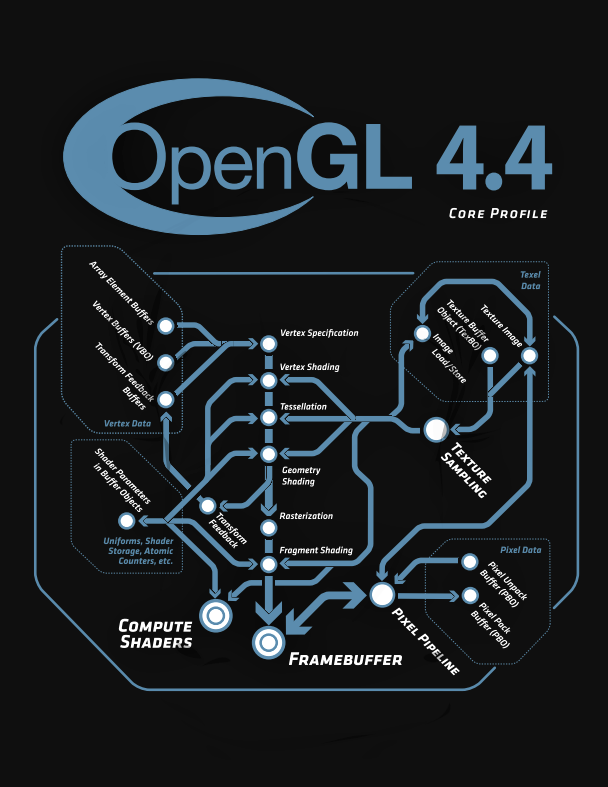#### Howdy, Stranger!

We are about to switch to a new forum software. Until then we have removed the registration on this forum.

#### In this Discussion

edited May 2017

Sometimes i find functions and workarounds still valid dated back in Processing Forum v1.0

• Here you can post everything you find interesting about Shaders, GLSL and Processing
Lost &Found Github Repository, general Links, Tutorials

#### Center

###### Plug your formula in and see what happens next
``````//imagine a question mark behind every line

void setup() {
size(640, 360, P2D);

noStroke();

new String[]{"#version 150 \n"
+ "in vec2 position;"
+ "void main() {"
+ "gl_Position = vec4(position.xy,0.,1.);"
+ "}"
}, new String[]{"#version 150 \n"
+ "out vec4 fragColor;"
+ "uniform vec3 iResolution;"
+ "void main() {"
+ "vec2 uvv = (gl_FragCoord.xy*2.-iResolution.xy)/iResolution.z;"
+ "float cir=.2/length(uvv);"
+ "fragColor = vec4(vec3(cir),1.);"
+"}"
});

shdr.set("iResolution", new PVector(width, height, Math.min(width, height)));

}

void draw() {
background(0);
rect(0, 0, width, height);
}
``````

• edited May 2017

#### observation

##### missing pixel
###### what is wrong with gl_FragCoord ?

this shader works around the world, - its a processing intern issue

##### the shader gives the corect results

so anyone who came along this post in the further, you have to convert from float into bytes or visa versa.

``````//Debuging shader with numbers

void setup() {
size(640, 360, P2D);

noStroke();

new String[]{"#version 150 \n"
+ "in vec2 position;"
+ "void main() {"
//NOTE I shift here in P2D mode (orthocam,viewport)
+ "gl_Position = vec4(position.xy-1,0.,1.);"
+ "}"
}, new String[]{"#version 150 \n"
+ "out vec4 fragColor;"
+ "uniform vec3 iResolution;"
+ "uniform vec3 iMouse;"
+ "uniform vec3 iDate;"
// Smaller Number Printing - <a href="/two/profile/P_Malin">@P_Malin</a>
// Creative Commons CC0 1.0 Universal (CC-0)
+"float DigitBin(const in int x){"
+   " return x==0?480599.0:x==1?139810.0:x==2?476951.0:x==3?476999.0:x==4?350020.0:x==5?464711.0:x==6?464727.0:x==7?476228.0:x==8?481111.0:x==9?481095.0:0.0;"
+"}"
+"float PrintValue(vec2 fragCoord, vec2 pixelCoord, vec2 fontSize, float value, float digits, float decimals) {"
+"  vec2 charCoord = (fragCoord - pixelCoord) / fontSize;"
+"  if(charCoord.y < 0.0 || charCoord.y >= 1.0) return 0.0;"
+"  float bits = 0.0;"
+"  float digitIndex1 = digits - floor(charCoord.x)+ 1.0;"
+"  if(- digitIndex1 <= decimals) {"
+"    float pow1 = pow(10.0, digitIndex1);"
+"    float absValue = abs(value);"
+"    float pivot = max(absValue, 1.5) * 10.0;"
+"    if(pivot < pow1) {"
+"      if(value < 0.0 && pivot >= pow1 * 0.1) bits = 1792.0;"
+"    } else if(digitIndex1 == 0.0) {"
+"      if(decimals > 0.0) bits = 2.0;"
+"    } else {"
+"      value = digitIndex1 < 0.0 ? fract(absValue) : absValue * 10.0;"
+"      bits = DigitBin(int (mod(value / pow1, 10.0)));"
+"    }"
+"  }"
+"  return floor(mod(bits / pow(2.0, floor(fract(charCoord.x) * 4.0) + floor(charCoord.y * 5.0) * 4.0), 2.0));"
+"}"
// Multiples of 4x5 work best
+"vec2 fontSize = vec2(4,5) * vec2(5,3);"
+"vec2 grid(int x, int y) { return fontSize.xx * vec2(1,ceil(fontSize.y/fontSize.x)) * vec2(x,y) + vec2(2); }"
+"vec2 limitTo(vec2 point, int x1, int y1, int x2, int y2, int startX, int startY) {"
+"    return clamp(point, grid(x1,y1), iResolution.xy - grid(x2,y2)) + grid(startX, startY);"
+"}"
+"void mainImage( out vec4 fragColor, in vec2 fragCoord ) {"
+"  vec3 vColour = vec3(0.0);"
+"  vec2 mouse = iMouse.xy;"
// Print Date
+  "vColour = mix( vColour, vec3(1.0, 1.0, 0.0), PrintValue(fragCoord, grid(0,0), fontSize, iDate.x, 5.0, 0.0));"
+ " vColour = mix( vColour, vec3(1.0, 1.0, 0.0), PrintValue(fragCoord, grid(5,0), fontSize, iDate.y , 2.0, 0.0));"
+  "vColour = mix( vColour, vec3(1.0, 1.0, 0.0), PrintValue(fragCoord, grid(8,0), fontSize, iDate.z, 2.0, 0.0));"
// Plot Mouse Pos
+"  float fDistToPointB = length( vec2(mouse.x, mouse.y) - fragCoord.xy) - 4.0;"
+"  vColour = mix(vColour, vec3(0.0, 1.0, 0.0), (1.0 - clamp(fDistToPointB, 0.0, 1.0)));"
// Print Sin Value
+"float fDecimalPlaces=0.;"
+"  if(mouse.x >= 0.0) {"
// Print Mouse X
+"    vec2 vPixelCoord2 = limitTo(mouse.xy, 8,2,8,4,-8,1);"
+"    float fValue2 = mouse.x / iResolution.x;"
+"    float fDigits = 0.0;"
+"    fDecimalPlaces = 5.0;"
+"    float fIsDigit2 = PrintValue(fragCoord, vPixelCoord2, fontSize, fValue2, fDigits, fDecimalPlaces);"
+"    vColour = mix( vColour, vec3(0.0, 1.0, 0.0), fIsDigit2);"
// Print Mouse Y
+"    vec2 vPixelCoord3 = limitTo(mouse.xy, 8,2,8,4,0,1);"
+"    float fValue3 = mouse.y / iResolution.y;"
+"    fDigits = 1.0;"
+"    float fIsDigit3 = PrintValue(fragCoord, vPixelCoord3, fontSize, fValue3, fDigits, fDecimalPlaces);"
+"    vColour = mix( vColour, vec3(0.0, 1.0, 0.0), fIsDigit3);"
+"}"
+"  fragColor = vec4(vColour,1.0);"
+"}"
+ "void main() {"
+ "vec2 uvv = (gl_FragCoord.xy*2.-iResolution.xy)/iResolution.z;"
+ "float cir=.2/length(uvv);"
//txutxi.com/?p=182
+ "mainImage(fragColor, gl_FragCoord.xy);"

+ "fragColor += vec4(vec3(cir)/9.,1.);"
+"}"
});

shdr.set("iResolution", new PVector(width, height, Math.min(width, height)));

shdr.set("iDate", new PVector(year(), month(), day()));

}

void draw() {
background(0);
println(map(mouseX, 0, width, 0, 1));

//shdr.set("iMouse", map(mouseX, 0, width, 0, 1), map(mouseY, 0, height, 1, 0),0);

shdr.set("iMouse", new PVector(mouseX,mouseY,0));
rect(0, 0, width, height);
}

/*new String[]{"#version 150 \n"
+ "in vec4 position;"
+ "uniform mat4 transformMatrix;"
+ "void main() {"
+ "gl_Position = transformMatrix * position;"
+ "}"
}*/
``````
• edited June 2017

# OKAY THIS WENT SOLVED

https://forum.processing.org/two/discussion/22385/reaction-diffusion-using-glsl-works-different-from-normal#latest• So simply put - don't rely on shaders for delicate computations and use them only for what they're meant - graphic effects.

• edited June 2017

# 23 Jan 2016

• edited June 2017

# PURE ART

## The OpenGL 4.4 Specification Cover Page

##### unfortunately nothing for mac users

https://www.khronos.org/registry/OpenGL/specs/gl/glspec44.core.pdf